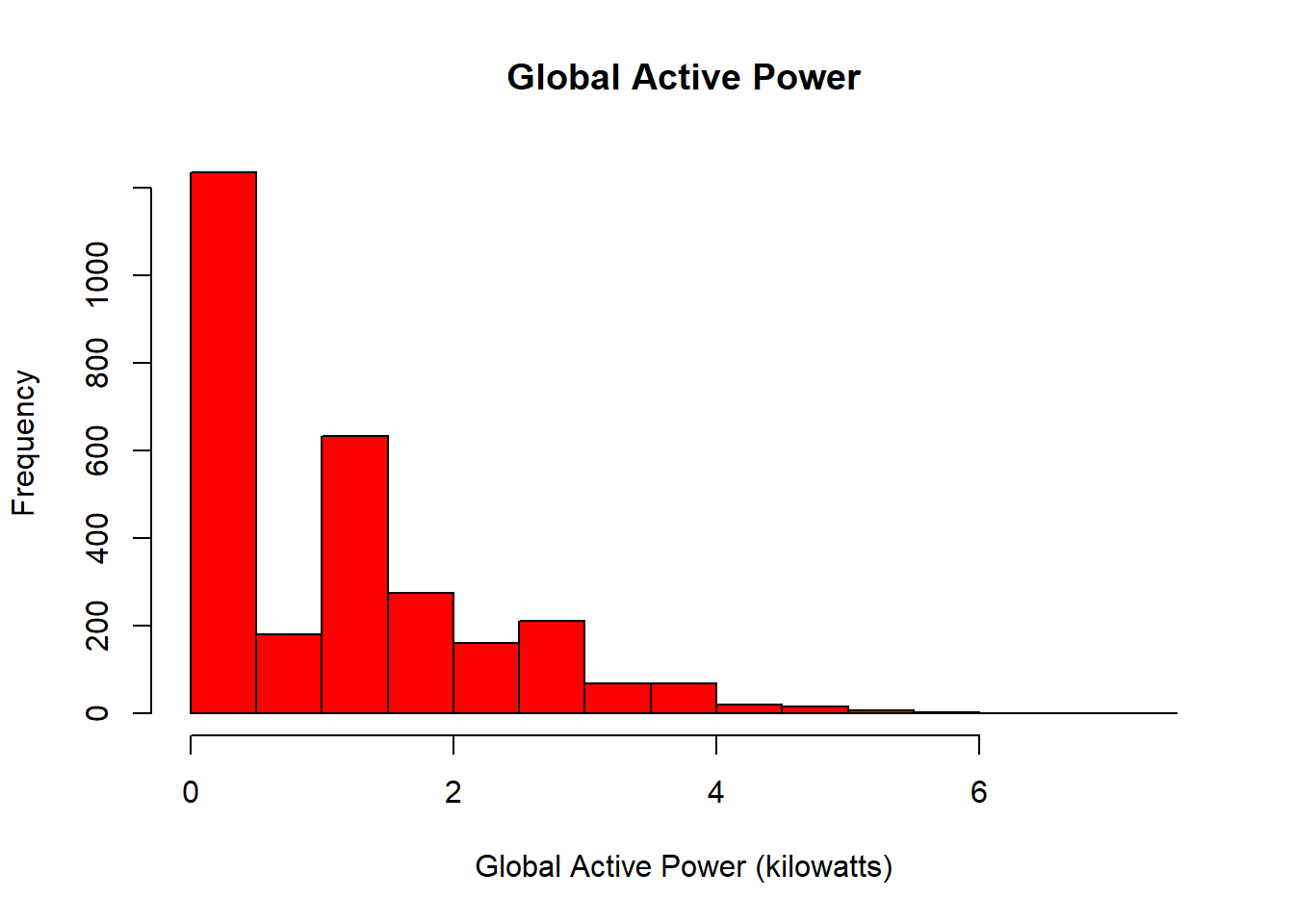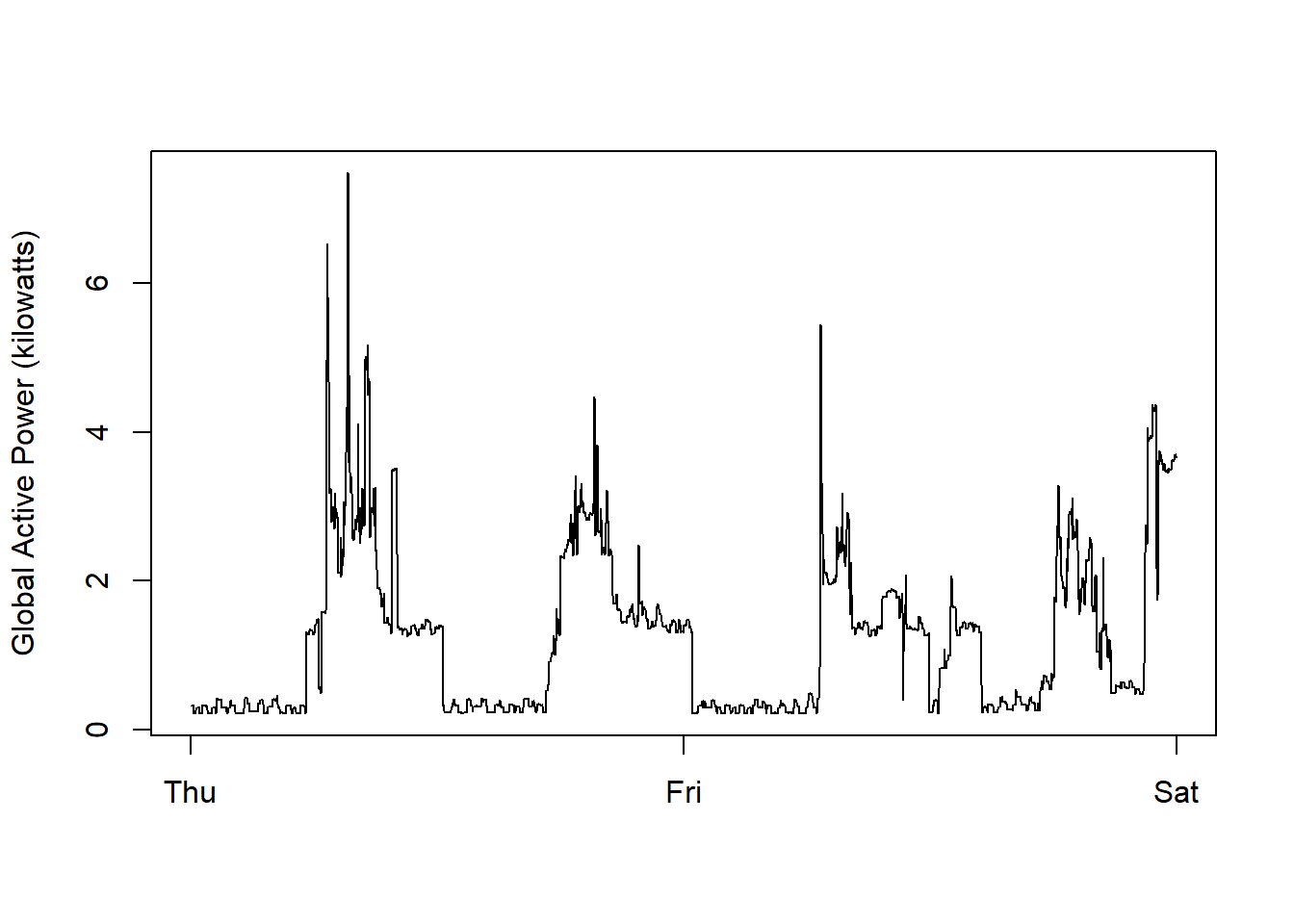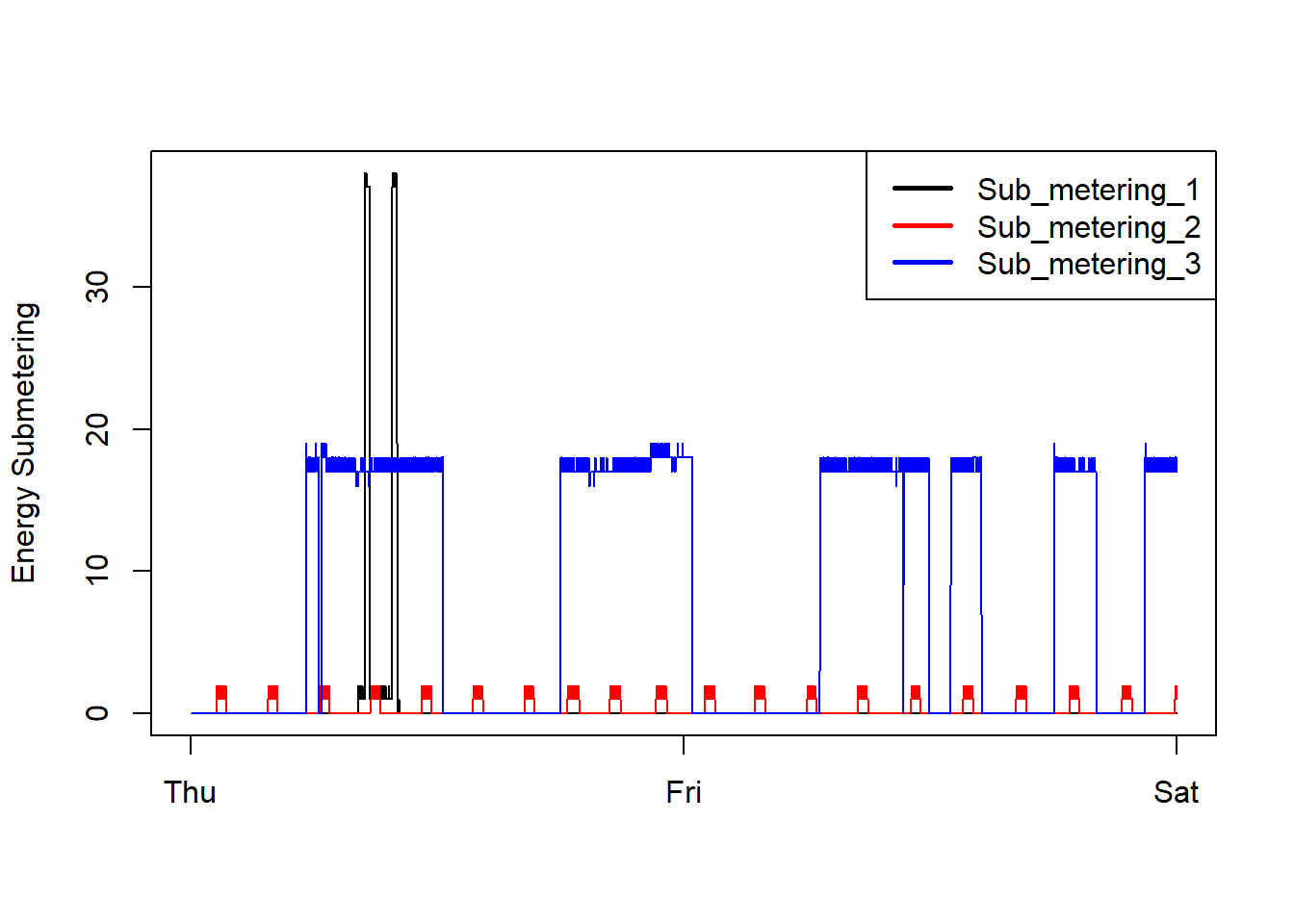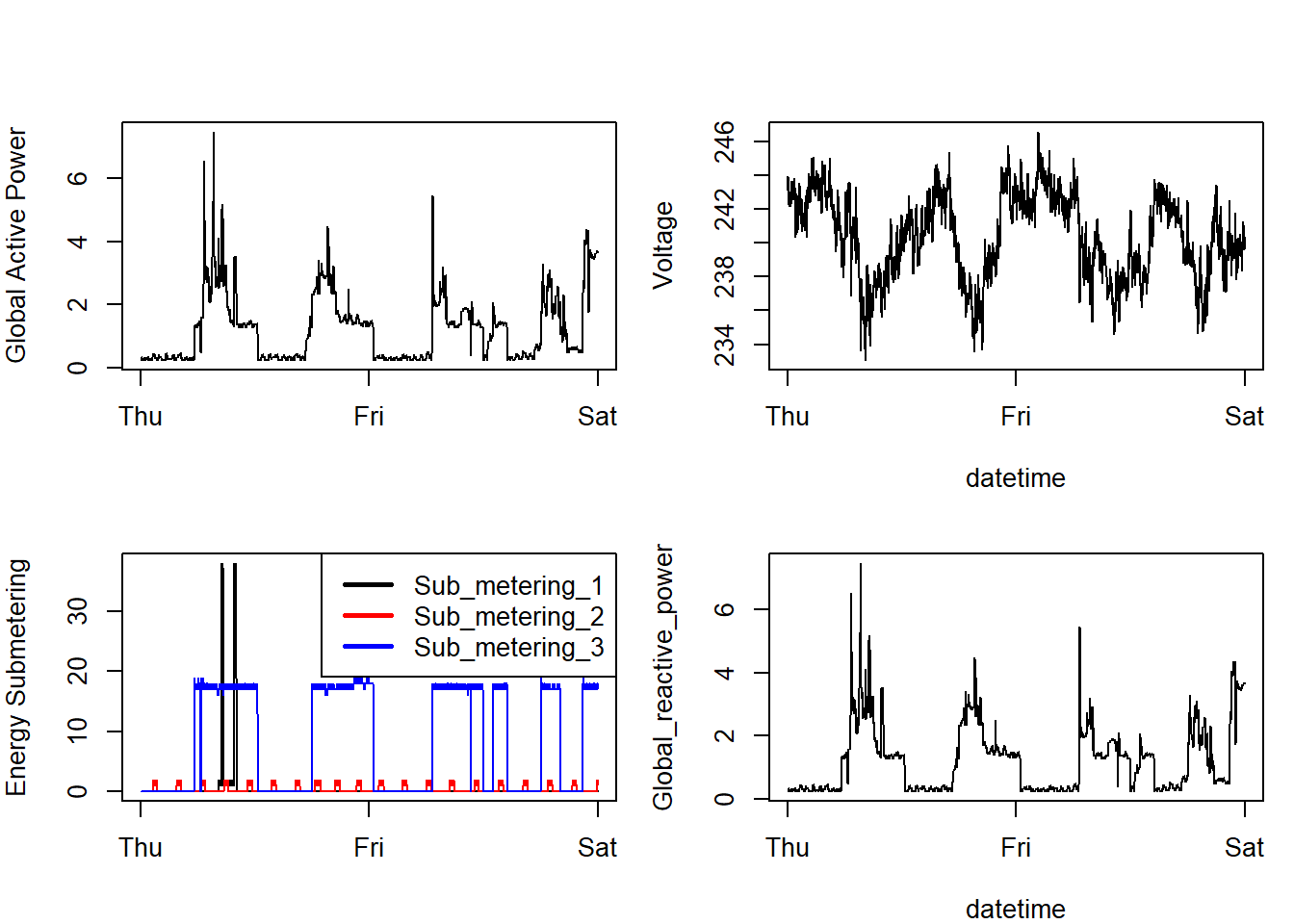## Introduction

This assignment uses data from the UC Irvine Machine Learning Repository, a popular repository for machine learning datasets. In particular, we will be using the “Individual household electric power consumption Data Set”.

### Description :

Measurements of electric power consumption in one household with a one-minute sampling rate over a period of almost 4 years. Different electrical quantities and some sub-metering values are available

The following descriptions of the 9 variables in the dataset are taken from the UCI web site:

Date: Date in format dd/mm/yyyy Time: time in format hh:mm:ss Global_active_power: household global minute-averaged active power (in kilowatt) Global_reactive_power: household global minute-averaged reactive power (in kilowatt) Voltage: minute-averaged voltage (in volt) Global_intensity: household global minute-averaged current intensity (in ampere) Sub_metering_1: energy sub-metering No. 1 (in watt-hour of active energy). It corresponds to the kitchen, containing mainly a dishwasher, an oven and a microwave (hot plates are not electric but gas powered). Sub_metering_2: energy sub-metering No. 2 (in watt-hour of active energy). It corresponds to the laundry room, containing a washing-machine, a tumble-drier, a refrigerator and a light. Sub_metering_3: energy sub-metering No. 3 (in watt-hour of active energy). It corresponds to an electric water-heater and an air-conditioner.

1- The dataset has 2,075,259 rows and 9 columns. First a rough estimate of how much memory the dataset will require in memory before reading into R should be calculated. Computer should have enough memory (most modern computers should be fine).

2- We will only be using data from the dates 2007-02-01 and 2007-02-02. One alternative is to read the data from just those dates rather than reading in the entire dataset and subsetting to those dates.

3- It may be useful to convert the Date and Time variables to Date/Time classes in R using the strptime() and as.Date() functions.

4- In this dataset missing values are coded as ?.

data <- read.table("Data.txt", header= TRUE, sep=";", stringsAsFactors=FALSE, dec=".")
summary(data)
##      Date               Time           Global_active_power
##  Length:2075259     Length:2075259     Length:2075259
##  Class :character   Class :character   Class :character
##  Mode  :character   Mode  :character   Mode  :character
##
##
##
##
##  Global_reactive_power   Voltage          Global_intensity
##  Length:2075259        Length:2075259     Length:2075259
##  Class :character      Class :character   Class :character
##  Mode  :character      Mode  :character   Mode  :character
##
##
##
##
##  Sub_metering_1     Sub_metering_2     Sub_metering_3
##  Length:2075259     Length:2075259     Min.   : 0.000
##  Class :character   Class :character   1st Qu.: 0.000
##  Mode  :character   Mode  :character   Median : 1.000
##                                        Mean   : 6.458
##                                        3rd Qu.:17.000
##                                        Max.   :31.000
##                                        NA's   :25979

### Creating Data Subset

Subset the data from the dates 2007-02-01 and 2007-02-02.

subsetdata <- data[data$Date %in% c("1/2/2007","2/2/2007"),] GlobalActivePower <- as.numeric(subsetdata$Global_active_power)
GlobalReactivePower <- as.numeric(subsetdata$Global_reactive_power) voltage <- as.numeric(subsetdata$Voltage)
subMetering1 <- as.numeric(subsetdata$Sub_metering_1) subMetering2 <- as.numeric(subsetdata$Sub_metering_2)
subMetering3 <- as.numeric(subsetdata$Sub_metering_3) ### Histogram we create histogram of our data as follows hist(GlobalActivePower, col="red", main="Global Active Power", xlab="Global Active Power (kilowatts)")### Time series plot we create time series plot for our dataset as follows timeseries <- strptime(paste(subsetdata$Date, subsetdata\$Time, sep=" "), "%d/%m/%Y %H:%M:%S")
plot(timeseries, GlobalActivePower, type="l", xlab="", ylab="Global Active Power (kilowatts)")### sub metering plots

plot(timeseries, subMetering1, type="l", ylab="Energy Submetering", xlab="")
lines(timeseries, subMetering2, type="l", col="red")
lines(timeseries, subMetering3, type="l", col="blue")
legend("topright", c("Sub_metering_1", "Sub_metering_2", "Sub_metering_3"), lty=1, lwd=2.5, col=c("black", "red", "blue"))### multiple plot

par(mfrow = c(2,2), mar = c(4,4,2,1), oma = c(0,0,2,0))
# First plot
plot(timeseries, GlobalActivePower, type="l", xlab="", ylab="Global Active Power", cex=0.2)
# Second plot
plot(timeseries, voltage, type="l", xlab="datetime", ylab="Voltage")
# Third plot
plot(timeseries, subMetering1, type="l", ylab="Energy Submetering", xlab="")
lines(timeseries, subMetering2, type="l", col="red")
lines(timeseries, subMetering3, type="l", col="blue")
legend("topright", c("Sub_metering_1", "Sub_metering_2", "Sub_metering_3"), lty=, lwd=2.5, col=c("black", "red", "blue"), bty="o")
# Fourth plot
plot(timeseries, GlobalActivePower, type="l", xlab="datetime", ylab="Global_reactive_power", cex=0.2)